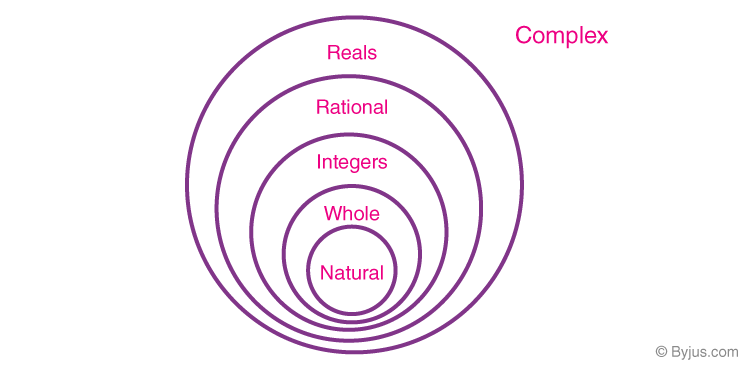# Rational Number Between Two Rational NumbersA rational number is a number which can be written in the form of

$$\begin{array}{l}p/q\end{array}$$
(ratio) where the denominator(q) is not equal to zero. This implies that it can be represented in the form of a fraction. Thus, every rational number has a numerator and a denominator, that is, one integer divided by another integer, where the denominator is not equal to zero.Let us first go through some of the examples and then learn how to find rational numbers between two other rational numbers.

## Finding Rational Numbers between Two Rational Number

• The simplest method to find a rational number between two rational numbers x and y is to divide their sum by 2.

Example:

$$\begin{array}{l}\frac{3}{1}~and~\frac{4}{1}\end{array}$$
. The rational number between them is (3 + 4)/2 =
$$\begin{array}{l}\frac{3+4}{2}=\frac{7}{2} \end{array}$$

Example: For numbers with common denominator like

$$\begin{array}{l}\frac{3}{20}~and~\frac{9}{20}\end{array}$$
, the rational number between them can be
$$\begin{array}{l}\frac{4}{20},~ \frac{5}{20},~\frac{6}{20},~\frac{7}{20},~\frac{8}{20}\end{array}$$
, etc.

• For a negative and a positive number like
$$\begin{array}{l}\frac{-3}{20}~and~\frac{3}{20}\end{array}$$
, we have
$$\begin{array}{l}\frac{-2}{20},~ \frac{-1}{20},~\frac{0}{20},~\frac{1}{20},~\frac{2}{20}\end{array}$$
, etc.
• We can write
$$\begin{array}{l}\frac{3}{20}\end{array}$$
as
$$\begin{array}{l}\frac{30}{200}\end{array}$$
and
$$\begin{array}{l}\frac{9}{20}\end{array}$$
as
$$\begin{array}{l}\frac{90}{200}\end{array}$$
. Thus, rational number between
$$\begin{array}{l}\frac{3}{20}~and~\frac{9}{20}\end{array}$$
can also be
$$\begin{array}{l}\frac{31}{200},~ \frac{32}{200},~\frac{33}{200},~\frac{34}{200},……..~\frac{89}{200}\end{array}$$
and so on .
• For finding rational numbers between two rational numbers with different denominators, we first find their equivalent fraction with the same denominator and then find the rational number between them. For example, for finding numbers between
$$\begin{array}{l}\frac{-1}{3}~and~\frac{5}{9}\end{array}$$
. , we convert
$$\begin{array}{l}\frac{-1}{3}~to~\frac{-1\times 3}{3 \times 3}=\frac{-3}{9}\end{array}$$
. Now, we have
$$\begin{array}{l}\frac{-2}{9},~ \frac{-1}{9},~\frac{0}{9},~\frac{1}{9},~\frac{2}{9},~\frac{3}{9},~\frac{4}{9}\end{array}$$
between them.

Rational number form one of the major part of mathematics and one cannot deny the importance of mathematics in today’s world.

Test your Knowledge on Rational Number Between Two Rational Numbers